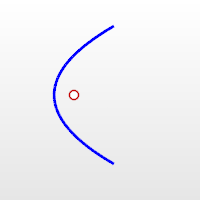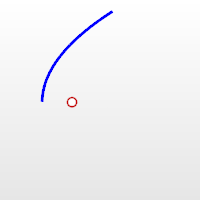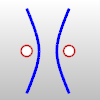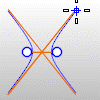# ParabolaCurve

Parabola >

<Options>

The Parabola command draws a parabolic curve.

#### Steps

• Follow the prompts for the selected option.
If no option is specified, the default is used.
Command-line options

MarkFocus

Places a point object at the focus location.Half

Draws only half the parabola.Focus

Lets you pick a focus point, direction, and end location.

#### Focus steps

1. Pick the focus.
2. Pick a direction.
This is the open direction.
3. Pick the end.
The focus to vertex distance and length of the parabola display at the command prompt.

Vertex

Lets you pick a vertex point, a focus point, and an end location.

#### Vertex steps

1. Pick the vertex.
2. Pick the focus.
This is the open direction.
3. Pick the end.
The focus to vertex distance and length of the parabola display at the command prompt.

# Parabola3PtThe Parabola3Pt command draws a parabolic curve through three picked points.

#### Steps

• Follow the prompts for the selected option.
If no option is specified, the default is used.
Command-line options
Mode

The Mode options specify the function of picked point that does not specify an endpoint.

##### Focus

The Focus option lets you pick two endpoints and a focus point.

##### ThroughPoint

The ThroughPoint option lets you pick two endpoints and a location for the parabola to pass through.

##### Vertex

The Vertex option lets you pick two end points and a vertex point.

PickOrder

##### Default

The Default option sets a start point, a second point that depends on the Mode setting, and then an endpoint.

##### EndsFirst

Specify the endpoints first and then the focus, throughpoint, or vertex mode.

##### EndsLast

Specify the focus, throughpoint, or vertex mode first and then the endpoints.

MarkFocus

Places a point object at the focus location.

# HyperbolaCurve

Hyperbola >

<Options>

The Hyperbola command draws a hyperbolic curve.

#### Steps

• Follow the prompts for the selected option.
Command-line options

Default
(Center)

The default option draws the hyperbola from the center, focus, and end.

#### Center steps

1. Pick the center.
2. Pick the focus.
3. Pick the end.

FromCoefficient

The FromCoefficient option uses the A and B coefficients of the hyperbola equation to define the curve.

#### FromCoefficient steps

1. Pick the center.
2. Pick a direction.
3. Pick the end.
##### A / B

These are the A and B coefficients of the equation that defines the hyperbola. The A coefficient represents the distance from the center point to the vertex of the hyperbola. If C is the distance from the center point to the foci of the hyperbola, then B2 = C2 - A2. The B coefficient can be considered the slope of the asymptotes.

FromFoci

The FromFoci option draws the hyperbola starting from the focus points.

#### FromFoci steps

1. Pick the first focus.
2. Pick the second focus.
3. Pick the end.

FromVertex

The FromVertex option draws the hyperbola starting from the vertices.

#### FromVertex steps

1. Pick the vertex.
2. Pick the focus.
3. Pick the end.

Drawing and display options

MarkFoci

Places point object at the focus locations.BothBranches

Both branches of the hyperbola are drawn.ShowAsymptotes

The asymptotes of the hyperbola are displayed as it is drawn.